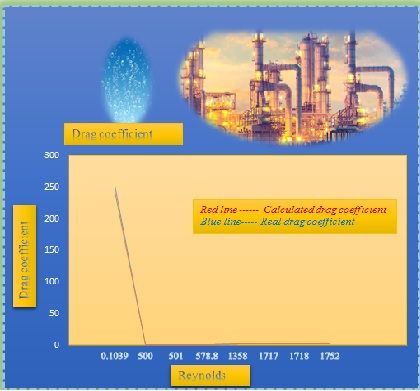# Review of drag coefficients on gas – liquid tower: the drag coefficient independent and dependent on bubble diameter in bubble column experiment

Document Type: Review Article

Author

Department of chemical Engineering, University of Sistan and Baluchestan, Zahedan, Iran

Abstract

Drag coefficient independent on bubble diameter is required to ease design sieve trays or bubble column, through simulation of computational fluid dynamic. In this paper, the drag coefficients, independent or dependent on the diameter, are reviewed for gas-liquid system. A number of drag coefficients are used for Computational Fluid Dynamics (CFD). Different forces are entered to the liquid-bubble separation surface in diverse directions. Forces are investigated with mathematical proving for Newtonian fluids and Eulerian coordinate. Finally, the external force as a new force, enter to the drag coefficient equations. Drag coefficient is included force coefficient. Drag force is entered in momentum equations. Drag coefficient is used in two-phase systems which bubbles and liquid are activated as dispersed and continuous phase, respectively. Bubbles and liquid are in contact with each other in separation surface on bubble. Drag force is created slip on separation surface. The drag coefficients are investigated depended on the size and configuration of bubbles. The drag coefficient of Krishna et al is used dependent on bubble diameter. Schiller - Nauman model drag coefficient is estimated with 9% error and dependence on bubble diameter. In this article, the modern drag coefficients are studied independent on the diameter and shape of the bubble. The Drag coefficients are resulted theoretical, mathematical and experimental independent and dependent of diameter bubble. The new Drag coefficient is presented dependent on surface tension and diameter of the tower hole with 6.3 of error approximately.

Graphical AbstractKeywords

### History

• Receive Date: 02 May 2019
• Revise Date: 11 June 2019
• Accept Date: 11 June 2019